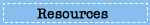# Starlight Response Page

In this 20-minute task, students look at a way of classifying stars based on their temperatures and luminosities. Students then compare data on two stars, and predict how characteristics like their temperature and luminosity might change throughout their lives.

# Question 1

List the numbers of the five stars you selected.

Describe the relationship between the masses of the stars you selected and their positions along the main sequence.

Describe the relationships between the masses of the stars you selected and their temperatures, radii, and luminosities.

Sample Complete Student Response:

The masses in the upper left of the chart are the highest and grow steadily smaller as you move to the bottom right of the chart. The higher the mass a star has the higher that the temperature is. Also the higher the mass of each star the higher the radii and the luminosities are.

#### Scoring Guide

This item was scored in three parts:

Part A - Selection of stars
Part B - Relationships between mass and position
Part C - Relationships between the mass and three variables

Part A

Complete:
Student response lists five stars that are spread out along the main sequence, including a minimum of one star numbered 1-4, one numbered 5-7, and one numbered 8-10.

Partial:
Student response lists three or four stars that are spread out along the main sequence, including a minimum of one star numbered 1-4, one numbered 5-7, and one numbered 8-10.

OR

Student response lists five stars that are not spread out along the main sequence.

Unsatisfactory/Incorrect:
Student response is inadequate or incorrect.

Part B

Complete:
Student response describes a correct relationship between mass and position along the main sequence. For example, the masses of the stars decrease moving from the upper left to the lower right part of the diagram.

Partial:
Student response provides a partially correct description between mass and position, such as a correct description of the relationship between mass and either the temperature or the luminosity of stars in the sequence.

Unsatisfactory/Incorrect:
Student response is inadequate or incorrect.

Part C

#### Scoring Guide

Complete:
Student response correctly describes the relationships between the masses of the stars and their temperatures, radii, and luminosities: as the mass of the star decreases, the temperature, radius, and luminosity decrease.

Essential:
Student response correctly describes the relationships between the masses of the stars and two of the variables: temperature, radius, and luminosity.

Partial:
Student response correctly describes the relationships between the masses of the stars and one of the variables: temperature, radius, and luminosity.

Unsatisfactory/Incorrect:
Student response is inadequate or incorrect.

Composite Score:

Student response received one of five possible composite scores (Complete, Satisfactory, Essential, Partial, Unsatisfactory/Incorrect) based on the student's combined performance on Parts A, B, and C of the item. For example, a student response Complete for Part A, Complete for Part B, and Essential for Part C received a composite score of Satisfactory.

Composite Score

Part A

Part B

Part C

Complete

Complete

Complete

Complete

Satisfactory

Complete

Complete

Essential

Complete

Partial

Complete

Partial

Complete

Complete

Complete

Partial

Essential

Partial

Complete

Essential

Partial

Partial

Complete

Complete

Complete

Partial

Complete

Unsatisfactory/Incorrect

Complete

Unsatisfactory/Incorrect

Complete

Complete

Essential

Complete

Partial

Partial

Partial

Complete

Partial

Complete

Complete

Unsatisfactory/Incorrect

Complete

Unsatisfactory/Incorrect

Essential

Unsatisfactory/Incorrect

Complete

Essential

Partial

Unsatisfactory/Incorrect

Complete

Unsatisfactory/Incorrect

Partial

Complete

Partial

Partial

Essential

Partial

Partial

Partial

Complete

Unsatisfactory/Incorrect

Partial

Complete

Partial

Unsatisfactory/Incorrect

Partial

Complete

Unsatisfactory/Incorrect

Unsatisfactory/Incorrect

Complete

Partial

Partial

Unsatisfactory/Incorrect

Essential

Unsatisfactory/Incorrect

Partial

Essential

Unsatisfactory/Incorrect

Unsatisfactory/Incorrect

Complete

Partial

Partial

Partial

Unsatisfactory/Incorrect

Partial

Unsatisfactory/Incorrect

Partial

Unsatisfactory/Incorrect

Partial

Partial

Complete

Unsatisfactory/Incorrect

Unsatisfactory/Incorrect

Unsatisfactory/Incorrect

Complete

Unsatisfactory/Incorrect

Unsatisfactory/Incorrect

Unsatisfactory/Incorrect

Essential

Partial

Unsatisfactory/Incorrect

Unsatisfactory/Incorrect

Unsatisfactory/Incorrect

Partial

Unsatisfactory/Incorrect

Unsatisfactory/Incorrect

Unsatisfactory/Incorrect

Partial

Unsatisfactory/Incorrect

Unsatisfactory/Incorrect

Unsatisfactory/Incorrect

Unsatisfactory/Incorrect

Percentage of twelfth-grade students in each response category: 2009
Complete Satisfactory Essential Partial Unsatisfactory/Incorrect Omitted
7 23 18 47 3 1
NOTE: Detail may not sum to totals because of rounding.

# Question 2

Your next task is to examine data on two stars, Star X and our Sun, which are currently on the main sequence.

The stars are plotted on the H-R diagram and data about them are shown in the table below the H-R diagram.
Use the information on the left and your knowledge of processes that occur in stars to predict what stages you expect each star to go through as it ages.

What future stages will the Sun likely go through? Select all that apply.

A. Giant
B. Supergiant
C. White dwarf
D. Planetary nebula
E. Supernova

Sample Complete Student Response: A, C, D

#### Scoring Guide

Complete:
Student response selects A, C, D and nothing else.

Essential:
Student response selects A, C and nothing else.

OR

Student response selects A, D and nothing else.

OR

Student response selects C, D and nothing else.

Partial:
Student response selects A and nothing else.

OR

Student response selects C and nothing else.

OR

Student response selects D and nothing else.

Unsatisfactory/Incorrect:
Student response is inadequate or incorrect.

Percentage of twelfth-grade students in each response category: 2009
Complete Essential Partial Unsatisfactory/Incorrect Omitted
# 5 37 57 #
# Rounds to zero.
NOTE: Detail may not sum to totals because of rounding.

# Question 3

What future stages will Star X likely go through? Select all that apply.

A. Giant
B. Supergiant
C. White dwarf
D. Planetary nebula
E. Supernova

Sample Complete Student Response: A, B, E

#### Scoring Guide

Complete:
Student response selects A, B, E and nothing else.

Essential:
Student response selects A, B and nothing else.

OR

Student response selects A, E and nothing else.

OR

Student response selects B, E and nothing else.

Partial:
Student response selects A and nothing else.

OR

Student response selects B and nothing else.

OR

Student response selects E and nothing else.

Unsatisfactory/Incorrect:
Student response is inadequate or incorrect.

Percentage of twelfth-grade students in each response category: 2009
Complete Essential Partial Unsatisfactory/Incorrect Omitted
3 22 42 31 1
NOTE: Detail may not sum to totals because of rounding.

# Question 4

As the animations show, the stages that Star X will go through as it ages are different than the stages that the Sun will go through as it ages.

Explain why this will happen. Use the data provided and your knowledge about stars to support your explanation.

Sample Complete Student Response:

The massive heat and gravity inside the star fuse two lighter elements together through nuclear fusion. This process releases heat and other energy, which keeps the temperature of the sun up and continues the cycle of fusion. The sun does not have enough heat and mass to produce O, Ne, Si or Fe.

####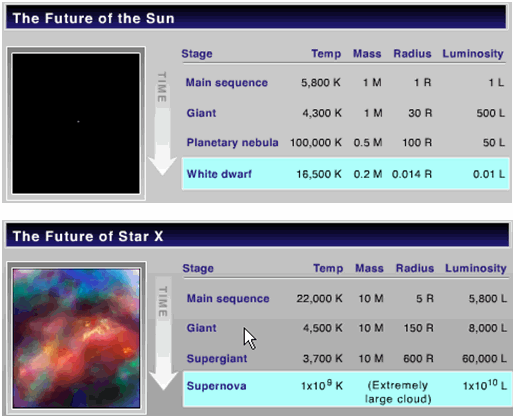Complete:
Student response indicates that the Sun and Star X go through different stages as they age because they have different masses. Response relates the greater mass of Star X to its supergiant (and/or supernova) stage(s) compared to the planetary nebula (and/or white dwarf) stage(s) of the Sun with its smaller mass.

Partial:
Student response recognizes that the mass of a star affects how it ages but the explanation does not specifically discuss the stage for each star and its relation to mass.

Unsatisfactory/Incorrect:
Student response is inadequate or incorrect.

Percentage of twelfth-grade students in each response category: 2009
Complete Partial Unsatisfactory/Incorrect Omitted
8 17 70 3
NOTE: Detail may not sum to totals because of rounding.

# Question 5

Some elements are produced only by Star X, and some elements are produced by both stars. How do the elements produced only by Star X differ from the elements produced by both stars?

Sample Complete Student Response:

The elements that were only produced by Star X had a larger mass than those produced by the Sun. Also, more protons and electrons were present in the elements produced by Star X then those of the sun. The Sun produced only Helium and Carbon, which are simpler elements. And Star X produced more complex elements.

####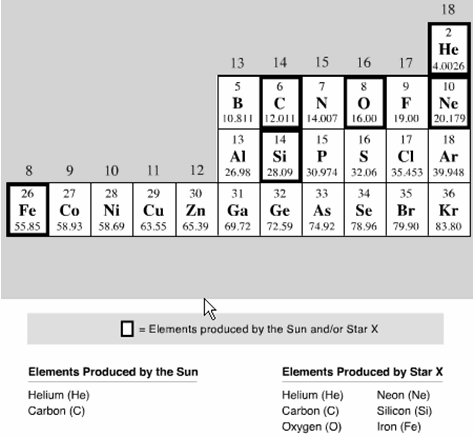Complete:
Student response describes a valid difference between the additional elements Oxygen (nonmetal), Neon (noble gas), Silicon (metalloid), Iron (metal) produced by Star X compared to the elements Helium (noble gas), Carbon (nonmetal) produced by both stars. For example, the additional elements have a greater mass than the elements produced by both stars.

Partial:
Student response describes a valid difference between some but not all of the additional elements compared to the elements produced by both the Sun and Star X. For example, the additional elements are larger than the elements produced by both stars.

Unsatisfactory/Incorrect:
Student response is inadequate or incorrect.

Percentage of twelfth-grade students in each response category: 2009
Complete Partial Unsatisfactory/Incorrect Omitted
14 5 76 2
NOTE: Detail may not sum to totals because of rounding.

# Question 6

Explain how the processes inside Star X at the supergiant stage produce oxygen, neon, silicon, and iron. Explain why the processes inside the Sun will not produce oxygen, neon, silicon, and iron.

Sample Essential Student Response:

In Star X there is a hige amount of pressure within the middle of the star. Here, lightler elements (like Hydrogen and helium) are forced together to form things like carbon, silicon, and iron. There is not enough pressure to force the fusion of elementary elements into more massive elements.

####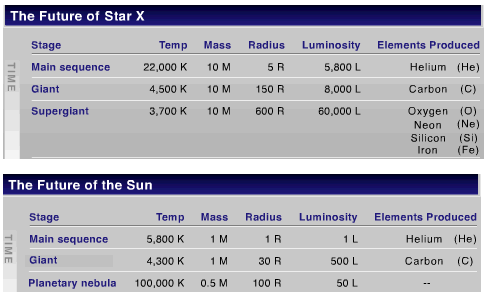Complete:
Student response provides a correct explanation that relates the differences of the condition in the cores of the stars that lead to the production of the heavier elements. The explanation consists of three parts:

• Indicates that the process of nuclear fusion of the lighter elements produces the heavier elements in Star X.
• Describes any two of the conditions in Star X compared to the Sun that make possible the fusion of the heavier elements from the lighter elements: greater mass; greater density; greater temperature (greater heat energy); greater pressure; greater gravitational force.

Essential:
Student response includes any two parts.

Partial:
Student response includes any one part.

Unsatisfactory/Incorrect:
Student response is inadequate or incorrect.

Percentage of twelfth-grade students in each response category: 2009
Complete Essential Partial Unsatisfactory/Incorrect Omitted
# 3 18 75 #
NOTE: Detail may not sum to totals because of rounding.

SOURCE: U.S. Department of Education, Institute of Education Sciences, National Center for Education Statistics, National Assessment of Educational Progress (NAEP), 2009 Science Assessment.

•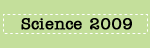•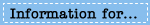•